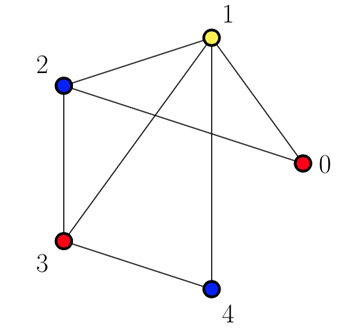# Understanding Graph Coloring (vertex): C++ code and visual graph in LaTeX (Part II)

- 3 mins

In the previous tutorial, we explored a very simple approach, step-by-step, to color a graph using the three colors available (blue, red and yellow).

Now we’ll put that approach into practice by using a C++ implementation (from the awesome guys at GeeksforGeeks) of that algorithm and my contribution to it, that was among others features, to generate a visualization of the graph colored by using the worldwide famous and open-source tool LaTeX and the powerful TikZ package.

### How to input the graph info: `input.txt`

One of the most important parts of the program is the method that actually colors the graph using the greedy approach.

However, before the `greedyColoring()` call, that actually colors the graph, the `input.txt` file is where you enter the information about your graph `G(V,E)`.

The first line is the number `V` of vertices and the following ones should contain the edges `E`. Let’s say we have the graph below:The input file should look like this:

``````5
0 1
0 2
1 2
1 3
1 4
2 3
3 4
``````

By doing so, the program will read that as `G(5, 7)` meaning your input graph has 5 vertices and 7 edges which connections are explicited in the lines.

### The `greedyColoring()` method

This method contains the main part of the code, which is the `result` array. This `result` array of size `V` is responsible for storing the final colors assigned to the vertices.

The first color assigned is going to be `0` and the algorithm assigns the remaining ones as it goes through the adjacency list.

As a result, the final `result` vector for the input graph illustrated here looks like `0, 1, 2, 0, 2` (5 vertices from 0-4) meaning that vertex 0 is colored in 0, vertex 1 is colored in 1, vertex 2 is colored in 2 and so on.

To handle the output in LaTeX, I created an array that chooses a real color based on the index, i.e., to get the color represented by the number:

Therefore color 0 is red, color 1 is yellow and color 2 is blue. The program replace them by the ones set in `key_value_colors`.

### `LaTeX/TikZ` code generated

By running the C++ code we get a `.tex` file that can be compiled to see the result of the algorithm graphically. It’s important to mention that in order to automatically see the `.pdf` you need to have LaTeX installed so the `pdflatex` command is recognized by the Terminal (Linux/Mac OS). Besides, the output for this example should be:Finally, in order to demonstrate how to use the program, I recorded this video where I show how to go from a regular graph as the input to the graph colored in a `.pdf` file as the output.